JMSLTM Numerical Library 7.2.0
com.imsl.stat

## Class LifeTables

• All Implemented Interfaces:
Serializable, Cloneable

```public class LifeTables
extends Object
implements Serializable, Cloneable```
Computes population (current) or cohort life tables based upon the observed population sizes at the middle (for population table) or the beginning (for cohort table) of some user specified age intervals. The number of deaths in each of these intervals must also be observed.

The probability of dying prior to the middle of the interval, given that death occurs somewhere in the interval, may also be specified. Often, however, this probability is taken to be 0.5. For a discussion of the probability models underlying the life table here, see the references.

Let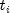, for i = 0, 1, ...,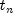denote the time grid defining the n age intervals, and note that the length of the age intervals may vary. Following Gross and Clark (1975, page 24), let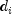denote the number of individuals dying in age interval i, where age interval i ends at time. For population table, the death rate at the middle of the interval is given by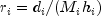, where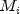is the number of individuals alive at the middle of the interval, and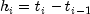,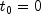. The number of individuals alive at the beginning of the interval may be estimated by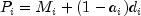where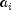is the probability that an individual dying in the interval dies prior to the interval midpoint. For cohort table,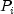is input directly while the death rate in the interval,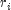, is not needed.

The probability that an individual dies during the age interval from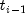tois given by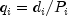. It is assumed that all individuals alive at the beginning of the last interval die during the last interval. Thus,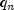= 1.0. The asymptotic variance of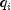can be estimated by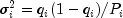For a population table, the number of individuals alive in the middle of the time interval (input in `nCohort[i]`) must be adjusted to correspond to the number of deaths observed in the interval. The algorithm assumes that the number of deaths observed in interval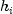occur over a time period equal to. Ifis measured over a period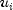, where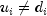, then `nCohort[i]` must be adjusted to correspond toby multiplication by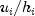, i.e., the valueinput as `nCohort[i]` is computed as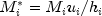Let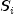denote the number of survivors at timefrom a hypothetical (for population table) or observed (for cohort table) population. Then,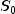``` =initialPopulation``` for population table, and`= nCohort` for cohort table, andis given by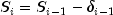where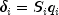is the number of individuals who die in the i th interval. The proportion of survivors in the interval is given by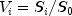while the asymptotic variance of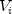can be estimated as follows: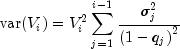The expected lifetime at the beginning of the interval is calculated as the total lifetime remaining for all survivors alive at the beginning of the interval divided by the number of survivors at the beginning of the interval. If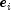denotes this average expected lifetime, then the variance ofcan be estimated as (see Chiang 1968)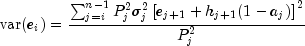where var(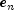) = 0.0.

Finally, the total number of time units lived by all survivors in the time interval can be estimated as: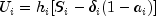Example, Serialized Form
• ### Constructor Summary

Constructors
Constructor and Description
```LifeTables(int[] nCohort, double[] age, double[] a)```
Constructs a new `LifeTables` instance.
• ### Method Summary

Methods
Modifier and Type Method and Description
`double[][]` `getLifeTable()`
Compute a cohort table.
`double[][]` `getPopulationTable(int[] nDeaths)`
Compute a population table.
`void` `setPopulationSize(int initialPopulation)`
Sets the population size at the beginning of the first age interval in requesting a population table.
• ### Methods inherited from class java.lang.Object

`clone, equals, finalize, getClass, hashCode, notify, notifyAll, toString, wait, wait, wait`
• ### Constructor Detail

• #### LifeTables

```public LifeTables(int[] nCohort,
double[] age,
double[] a)```
Constructs a new `LifeTables` instance. The number of classes, nClasses is equal to the length of the input array ```nCohort ```.
Parameters:
`nCohort` - an `int` array of length nClasses containing the cohort sizes during each interval. If the Population Table option is used, then ```nCohort[i] ``` contains the size of the population at the midpoint of interval `i`. Otherwise, ``` nCohort[i]``` contains the size of the cohort at the beginning of interval `i`. When requesting a population table, the population sizes in ```nCohort ``` may need to be adjusted to correspond to the number of deaths in `nDeaths`. See the class description section for more information.
`age` - a `double` array of length nClasses + 1 containing the lowest age in each age interval, and in ``` age[nClasses]```, the endpoint of the last age interval. Negative `age` indicates that the age intervals are all of length `|age|` and that the initial age interval is from 0.0 to `|age|`. In this case, all other elements of age need not be specified. ``` age[nClasses]``` need not be specified when getting a cohort table.
`a` - a `double` array of length nClasses containing the fraction of those dying within each interval who die before the interval midpoint. A common choice for all `a[i]` is 0.5. This choice may also be specified by setting ```a ``` to any negative value. In this case, the remaining values of `a` need not be specified.
• ### Method Detail

• #### getLifeTable

`public double[][] getLifeTable()`
Compute a cohort table.
Returns:
a `double` matrix of dimensions nClasses by 12 containing the population table. Entries in the ith row are for the age interval defined by `age[i]`. Column definitions are described in the following table.

 Column Description 0 Lowest age in the age interval. 1 Fraction of those dying within the interval who die before the interval midpoint. 2 Number surviving to the beginning of the interval. 3 Number of deaths in the interval. 4 Death rate in the interval. For cohort table, this column is set to NaN (not a number). 5 Proportion dying in the interval. 6 Standard error of the proportion dying in the interval. 7 Proportion of survivors at the beginning of the interval. 8 Standard error of the proportion of survivors at the beginning of the interval. 9 Expected lifetime at the beginning of the interval. 10 Standard error of the expected life at the beginning of the interval. 11 Total number of time units lived by all of the population in the interval.

• #### getPopulationTable

`public double[][] getPopulationTable(int[] nDeaths)`
Compute a population table.
Parameters:
`nDeaths` - an `int` array of nClasses containing the number of deaths in each age interval.
Returns:
a `double` matrix of dimensions nClasses by 12 containing the population table. Entries in the ith row are for the age interval defined by `age[i]`. Column definitions are the same as in `getLifeTable`.
• #### setPopulationSize

`public void setPopulationSize(int initialPopulation)`
Sets the population size at the beginning of the first age interval in requesting a population table. A default value of 10,000 is used to allow easy entry of `nCohorts` and `nDeaths` when numbers are available as percentages.
Parameters:
`initialPopulation` - an `int` scalar specifying the initial population. Default: ``` initialPopulation = 10000```.
JMSLTM Numerical Library 7.2.0